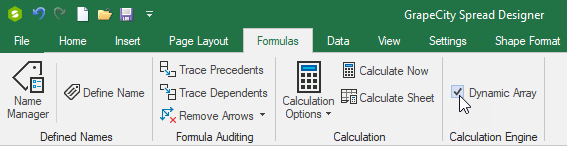﻿ Import and Export Spreadsheets with an External Workbook Reference | Spread# Import and Export Spreadsheets with an External Workbook Reference (Spread.NET WinForms)

This article describes how to use Spread.NET WinForms to create a Custom Function using a Dynamic Array to return the workbook's External Links in a cell range using a simple formula.

Here we will cover:

1. How to Import and Export Microsoft Excel Workbook (.XLSX or .XLS) or Macro-enabled Excel Workbook (*.XLSM) containing External Links (e.g. cell or range references) to other Workbooks in some Cell Formulas.
2. How to create a Custom Function GETLINKS() which uses IEvaluationContext.ReferenceSource to get a reference to the IWorkbook and then returns the External Links in a Cell Formula.
3. How to use the Dynamic Array feature to show this list of External Links in a Cell Range.

Spread.NET 13 WinForms supports external links to other workbooks like Microsoft Excel, and you can import existing workbooks that contain such external links:

#### [C#]

##### Open Excel File
``````OpenFileDialog d = new OpenFileDialog();
d.Filter = "Excel Workbook (*.XLSX;*.XLSM;*.XLS)|*.XLSX;*.XLSM;*.XLS";
d.Title = "Open Excel Workbook";
if (d.ShowDialog() == DialogResult.OK)
``````

#### [VB]

##### Open Excel File
``````Dim d As OpenFileDialog = New OpenFileDialog()
d.Filter = "Excel Workbook (*.XLSX;*.XLSM;*.XLS)|*.XLSX;*.XLSM;*.XLS"
d.Title = "Open Excel Workbook"
If d.ShowDialog() = DialogResult.OK Then FpSpread1.OpenExcel(d.FileName)
``````

When a workbook contains external links, then the IWorkbook.LinkSources property will return a string array containing the workbook filenames.

Managing these external links can become a problem when you have many related workbooks that reference one another, or many versions of the same workbook that might be linked. Using the Custom Function feature, you can extend the Calculation Engine to support a simple cell formula that returns a list of all external links in the workbook.

### Creating A Custom Function

Creating a Custom Function requires implementing a class with code to calculate the function and return the result to the Calculation Engine. A Custom Function inherits from the GrapeCity.CalcEngine.Function class and must specify the name, minimum number of arguments, maximum number of arguments, and FunctionAttributes to the base class constructor:

#### [C#]

``````sealed class GetLinksFunction : Function
{
{
}
...
``````

#### [VB]

``````NotInheritable Class GetLinksFunction
Inherits [Function]
Public Sub New()
End Sub
...
``````

This example defines a new function named GETLINKS which accepts no arguments and returns an array value.

To get the External Links, the function will need to use the IWorkbook interface, so the class implements a private helper function GetWorkbook which returns the IWorkbook from the IEvaluationContext:

#### [C#]

##### GetWorkbook function
``````...
private IWorkbook GetWorkbook(IEvaluationContext context)
{   // use IEvaluationContext.ReferenceSource to get the IWorkbook
IWorkbook workbook;
IWorksheet worksheet = context.ReferenceSource as IWorksheet;
if (worksheet != null)
{
workbook = worksheet.Workbook;
}
else
{
workbook = context.ReferenceSource as IWorkbook;
}
return workbook;
}
...
``````

#### [VB]

##### GetWorkbook function
``````...
Private Function GetWorkbook(context As IEvaluationContext) As IWorkbook
' Use IEvaluationContext.ReferenceSource to get the IWorkbook
Dim workbook As IWorkbook = Nothing
If TypeOf context.ReferenceSource Is IWorksheet Then
Dim worksheet As IWorksheet = CType(context.ReferenceSource, IWorksheet)
workbook = worksheet.Workbook
ElseIf TypeOf context.ReferenceSource Is IWorkbook Then
workbook = CType(context.ReferenceSource, IWorkbook)
End If
Return workbook
End Function
...
``````

A Custom Function must override the Evaluate method and implement the logic to calculate the function and return the calculated result. For the GETLINKS function, Evaluate will use the IWorkbook returned by GetWorkbook to get the External Links into a string array and return the array:

#### [C#]

``````...
protected override void Evaluate(IArguments args, IValue result)
{
IWorkbook workbook = GetWorkbook(args.EvaluationContext);
if (workbook != null && workbook.LinkSources != null && workbook.LinkSources.Length > 0)
{   // copy the link sources to array and return
for (int i = 0; i < links.Length; i++)
result.SetValue(array);
}
else // return #N/A error
result.SetValue(CalcError.NotAvailable);
}
``````

#### [VB]

``````...
Protected Overrides Sub Evaluate(args As IArguments, result As IValue)
Dim workbook As IWorkbook = GetWorkbook(args.EvaluationContext)
' copy the link sources to array and return
Dim array As IValuesArray = result.CreateValuesArray(links.Length, 1)
For i As Integer = 0 To links.Length - 1
Next
result.SetValue(array)
Else ' return #N/A error
result.SetValue(CalcError.NotAvailable)
End If
End Sub
End Class
``````

If there are one ore more External Links in the workbook, then the code will get the IWorkbook.LinkSources and set them into the new IValuesArray, then use result.SetValue to return the array to the Calculation Engine. Otherwise, the function returns the #N/A error value. For convenience, this function returns the array of values in a vertical array rather than a horizontal array (as the old Excel 4.0 LINKS macro would return it), since this is more useful.

### Using Dynamic Array to Spill Results

Using the new Dynamic Array feature, you can enable the custom GETLINKS function to spill results to adjacent cells and create a list of external links in a range of cells. To enable the Dynamic Array feature, you must either open the Spread Designer tool and check the box for Dynamic Array in the Formulas tab:Figure 1 - Enable the Dynamic Array feature using Spread Designer ribbon bar Formulas tab

Or you can enable the Dynamic Array feature in code:

#### [C#]

##### Enable Dynamic Arrays in Code
``````fpSpread1.AsWorkbook().WorkbookSet.CalculationEngine.CalcFeatures = GrapeCity.Spreadsheet.CalcFeatures.DynamicArray;
``````

#### [VB]

##### Enable Dynamic Arrays in Code
``````FpSpread1.AsWorkbook().WorkbookSet.CalculationEngine.CalcFeatures = GrapeCity.Spreadsheet.CalcFeatures.DynamicArray
``````

When the Dynamic Array feature is enabled, then formulas can return arrays of results and spill to adjacent empty cells. You can use the GETLINKS function to create a list of the external links in the workbook in a new worksheet and more conveniently manage those links in a spilled range.

##### Demo sample projects are available here: